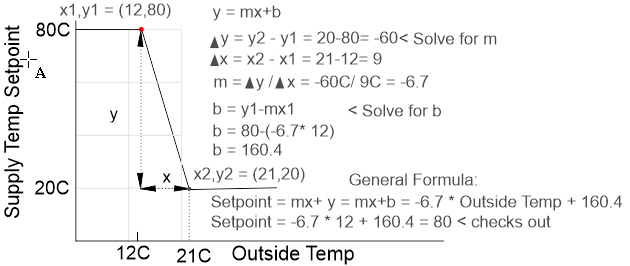# Outdoor Temperature Reset Example

Is there a function/syntax to map a number in one range into another range? Siemens call it a Table Syntax. TABLE(input,output,x1,y1,…,x7,y7) Whereas, input= Input Variable, Output=Output Variable, X1 & Y1, Pairs of coordinates that define the x-y relationship. y1 is the value of the output when the input equals x1; y2 is the value of the output when the input is x2, etc.

1 Like

Sorry we dont have a table function like that, you’d have to do a bunch of if this then that type program lines to accomplish that. Not very elegant I admit.

Hi Maurice,
Ok, so how do I write code for supply air reset, or outside temperature weather compensated control without a table statement, as its a sliding scale???

I can help with that sure. It’s just a formula. Give me a day.

Maurice

Linear equation: y=mx + b
Draw it out on a xy graph and solve for the slope: rise /run, then b by substitution of know coordinates at one end of your line. Then last prove your equation by solving for the other end of your line.
This works well for supply air, boiler water, whatever has a straight line equation.
I can help if you send me more details.

Sure, so what I’m trying to achieve is at outside temperature >21°C then flow temperature is 20°C and at outside temperature <12°C then flow temperature to be 80°C. So if the outside temperature is 16.5°C the flow temperature should be maintained at 50°C, about half way on the outside scale and so forth.

Here’s a graph of setpoint versus outside air temp and the basic middle school math that drives it. That took me way longer than it should have…!Here’s a general formula in control basic. Most of these variables can stay as local variables so they appear grey in the control basic editor. The SETPOINT is a variable which we’ll show on graphics displays, use in the programs and PID loops so it is set up in the VARS table and therefore shows as blue in the editor at Tab1. Similarly the outside air temperature is defined in the inputs table at Tab2 and also shows as blue in the editor.

Set the outside air temperature to MANUAL mode at Tab3 and watch the setpoint follow the formula, it checks out OK at both the max and the min outside temperature.

…and good thing I did that test, we need to clip the setpoint at the min and max values, added that in line 140.

10 REM **** OUTDOOR AIR RESET FOR HOT WATER SUPPLY TEMP *******
20 REM CHANGE THESE NUMBERS TO SUIT YOUR PROJECT
30 REM X1,Y1 ARE THE OUTSIDE TEMP AND SETPOINT RESPECTIVELY AT OAT=12C
40 REM X2,Y2 ARE THE OUTSIDE TEMP AND SETPOINT RESPECTIVELY AT OAT=20C
50 XONE = 12
60 YONE = 80
70 XTWO = 21
80 YTWO = 20
90 RISE = YTWO - YONE
100 RUN = XTWO - XONE
110 SLOPE = RISE / RUN
120 BINT = YONE - SLOPE * XONE
130 SETPOINT = SLOPE * OUTSIDE + BINT
140 SETPOINT = MIN( YONE , MAX( SETPOINT , YTWO ) )

Yours is way prettier!

Hi Maurice, Nice. How do I put this into code, controlling a 0-10Vdc output (Mixing Valve)?

Ok, looks good, I will do my own testing. Thanks for the help.

1 Like

I did an example of PID control the other day, here’s a link. If you have trouble you can post here.

1 Like

2 posts were split to a new topic: Settign up expansion IO on T3 controllers

Bringing up an old post with a different take on this.
This is how I code outdoor reset -
ACTIVE SETPOINT = Y2+(Y1-Y2)*(OAT-X2)/(X1-X2)
IF ACTIVE SETPOINT < Y2 THEN ACTIVE SETPOINT = Y2
IF ACTIVE SETPOINT > Y1 THEN ACTIVE SETPOINT = Y1

My program example:
50 REM *** RESET SCHEDULE***
60 VAR15 = VAR13 + ( VAR14 - VAR13 ) * ( OAT - VAR12 ) / ( VAR11 - VAR12 )
70 IF VAR15 < VAR13 THEN VAR15 = VAR13
80 IF VAR15 > VAR14 THEN VAR15 = VAR14